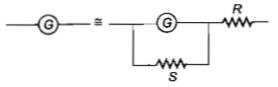NEET Questions Solved

NEET - 2011

A galvanometer of resistance, G is shunted by a resistance S ohm. To keep the main current in the circuit unchanged, the resistance to be put in series with the galvanometer is

(a) $\frac{{S}^{\mathit{2}}}{\left(S+G\right)}$                                             (b) $\frac{SG}{\left(S+G\right)}$

(c) $\frac{{G}^{\mathit{2}}}{\left(S+G\right)}$                                              (d) $\frac{G}{\left(S+G\right)}$

Current will be unchanged if resistance remains same so$G=\frac{\mathrm{GS}}{G+S}+R\phantom{\rule{0ex}{0ex}}R=G-\frac{GS}{G+S}\phantom{\rule{0ex}{0ex}}R=\frac{{G}^{\mathit{2}}}{G+S}$

Difficulty Level:

• 16%
• 39%
• 31%
• 16%